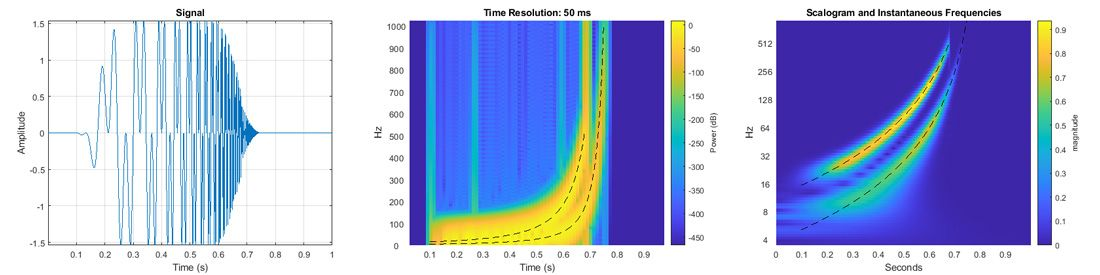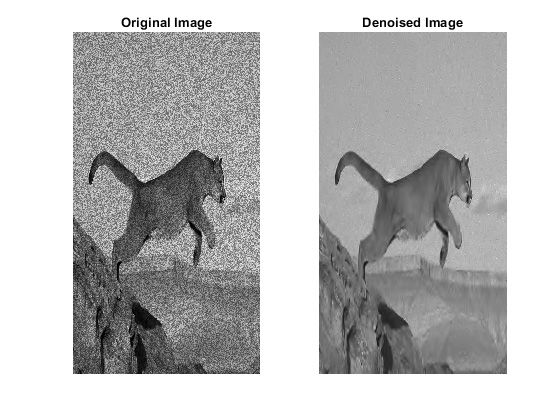# Wavelet Transforms in MATLAB

## Analyze signals and images in the wavelet domain

Wavelet transforms are mathematical tools for analyzing data where features vary over different scales. For signals, features can be frequencies varying over time, transients, or slowly varying trends. For images, features include edges and textures. Wavelet transforms were primarily created to address limitations of the Fourier transform.

While Fourier analysis consists of decomposing a signal into sine waves of specific frequencies, wavelet analysis is based on decomposing signals into shifted and scaled versions of a wavelet. A wavelet, unlike a sine wave, is a rapidly decaying, wave-like oscillation. This enables wavelets to represent data across multiple scales. Different wavelets can be used depending on the application. Wavelet Toolbox™ for use with MATLAB® supports Morlet, Morse, Daubechies, and other wavelets used in wavelet analysis.

Audio signals, time-series financial data, and biomedical signals typically exhibit piecewise smooth behavior punctuated by transients. Similarly, images typically include homogenous, piecewise smooth regions separated by transients, which appear as edges.  For both signals and images, the smooth regions and transients can be sparsely represented with wavelet transforms.

Capturing transient behavior in signals using a MATLAB wavelet transform.

Wavelet transforms can be classified into two broad classes: the continuous wavelet transform (CWT) and the discrete wavelet transform (DWT).

The continuous wavelet transform is a time-frequency transform, which is ideal for analysis of non-stationary signals. A signal being nonstationary means that its frequency-domain representation changes over time. CWT is similar to the short-time Fourier transform (STFT). The STFT uses a fixed window to create a local frequency analysis, while CWT tiles the time-frequency plane with variable-sized windows. The window widens in time, making it suitable for low-frequency phenomena, and narrows for high-frequency phenomena. The continuous wavelet transform can be used to analyze transient behavior, rapidly changing frequencies, and slowly varying behavior.Analyzing a hyperbolic chirp signal (left) with two components that vary over time in MATLAB. The short-time Fourier transform (center) does not clearly distinguish the instantaneous frequencies, but the continuous wavelet transform (right) accurately captures them. See the MATLAB code.

With the discrete wavelet transform scales are discretized more coarsely than with CWT. This makes DWT useful for compressing and denoising signals and images while preserving important features. You can use discrete wavelet transforms to perform multiresolution analysis and split signals into physically meaningful and interpretable components.Original (left) and denoised (right) images. The image was denoised while preserving the edges using a wavelet denoising function. See the MATLAB code.

For more information on applying wavelet techniques and selecting the right wavelets for your application in MATLAB, see Wavelet Toolbox.

See also: Signal Processing Toolbox, DSP System Toolbox, wavelet transforms videos, empirical mode decomposition, Denoising